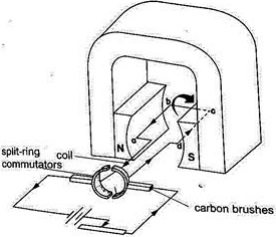# Magnetic effect of an electric current

### Guest Account

Attempt Form Two Physics Questions
Guest Account

# Magnetic Effect of an Electric Current

### Introduction: Oersted’s discovery

- Hans Christian Oersted discovered the magnetic effect of a current in 1819.
- The direction of the field is dependent on the direction of the current.

### Determining the direction of the lines of force

- The direction of the lines of force can be determined using a simple rule called the right-hand screw rule.
- This rule states that if a right-hand screw advances in the direction of the current, then the rotation of the screw is in the direction of the field.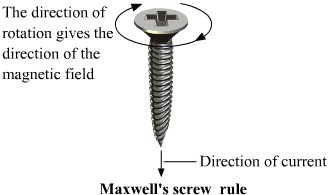- Another rule is the right-hand grip rule which states that if the wire carrying a current is gripped with the right hand, using the thumb along the conductor and pointing in the direction of the current, then the direction of curled fingers is in the direction of the lines of force.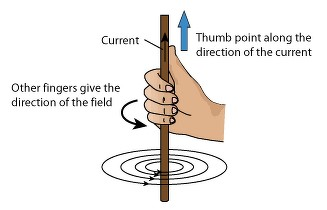### Magnetic Field Due to a Solenoid : The Rule for Polarity

- A solenoid is a cylindrical coil of wire acting as a magnet when carrying electric current.
- The direction of the field can be determined using a simple rule stated as follows if the coil (solenoid) is viewed from one end and the current flows in an anticlockwise direction at that end, then that end is the North Pole. If the current flows in a clockwise direction, then that end is the South Pole.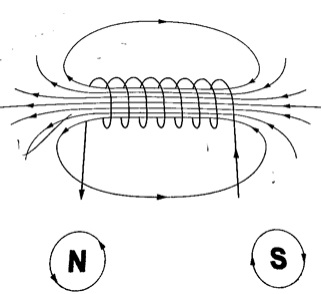### Electromagnets

- A n electromagnet is a soft metal core made into a magnet by passing an electric current through a coil surrounding it.
Factors Affecting the Strength of an Electromagnet
• Increasing current through the coil
• Increasing the number of turns of the coil
• Using iron of C- core shape which brings both magnetic poles together

## Some Applications of Electromagnets

### a) Electric Bell

- When the switch is closed the current passing through the solenoids magnetizes them and they pull the soft iron armature which makes the hammer hit the gong therefore producing sound.
- When the hammer hits the gong the contact between the spring and the screw is broken and then stops the current from flowing.
- The soft iron core loses its magnetism and releases the armature which is then pulled back by the screw.
- The contact between the spring and the screw is regained and the process repeats itself again and again therefore the gong is struck continuously.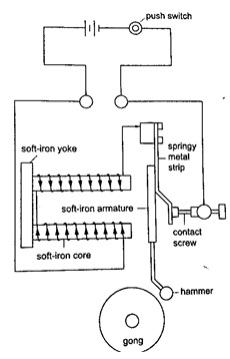- It consists of a u-magnet made by attaching two soft-iron bars to the end of a short permanent magnet.
- The solenoids are wound in opposite directions around the bars.
- When the phone is lifted the current flows through the solenoids depending on the microphone on the other end of the line.
- These varying current spasms induce magnetism of varying strengths in the iron bars which in turn causes the magnetic alloy diaphragm to vibrate differently producing sound.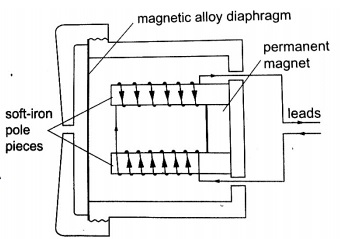### Force on a Current-Carrying Conductor in a Magnetic Field

- The factors affecting the magnitude of the force are:
• The current flowing in the conductor
• The strength of the magnet
• The length of the conductor in the magnetic field
- The relationship between the directions of the current, field and force are mutually perpendicular.
- They are summarized in a law called Fleming’s right-hand rule or the motor rule.
- This rule states that if you hold the first finger, the second finger and the thumb of your left hand mutually perpendicular to each other, so that the first finger points in the direction of the magnetic field and the second finger points in the direction of the current in the conductor, then the thumb points in the direction of the force acting on the conductor.### Applications of the Force on a Conductor

Simple D.C Motor
- Consists of a rectangular coil of wire mounted on an axle which can rotate between the poles of a magnet.
- For the rotation to be continuous the ends of the coil is connected to half-rings called the split-ring commutators.
- The battery terminals are attached to brushes which slide on these half-rings.
- D.C motors are useful as car starter motors, hand drills, machine motors, fans etc.